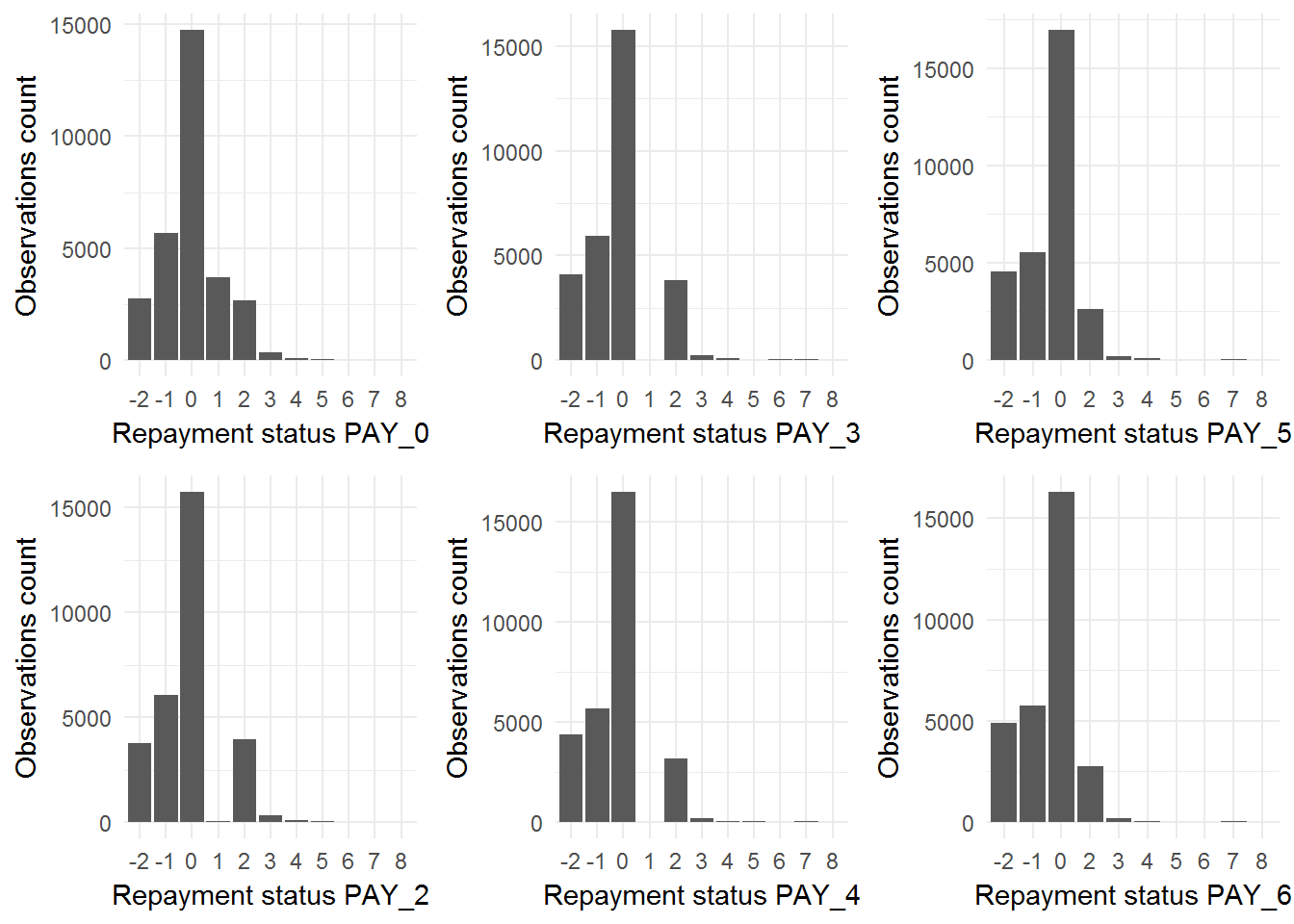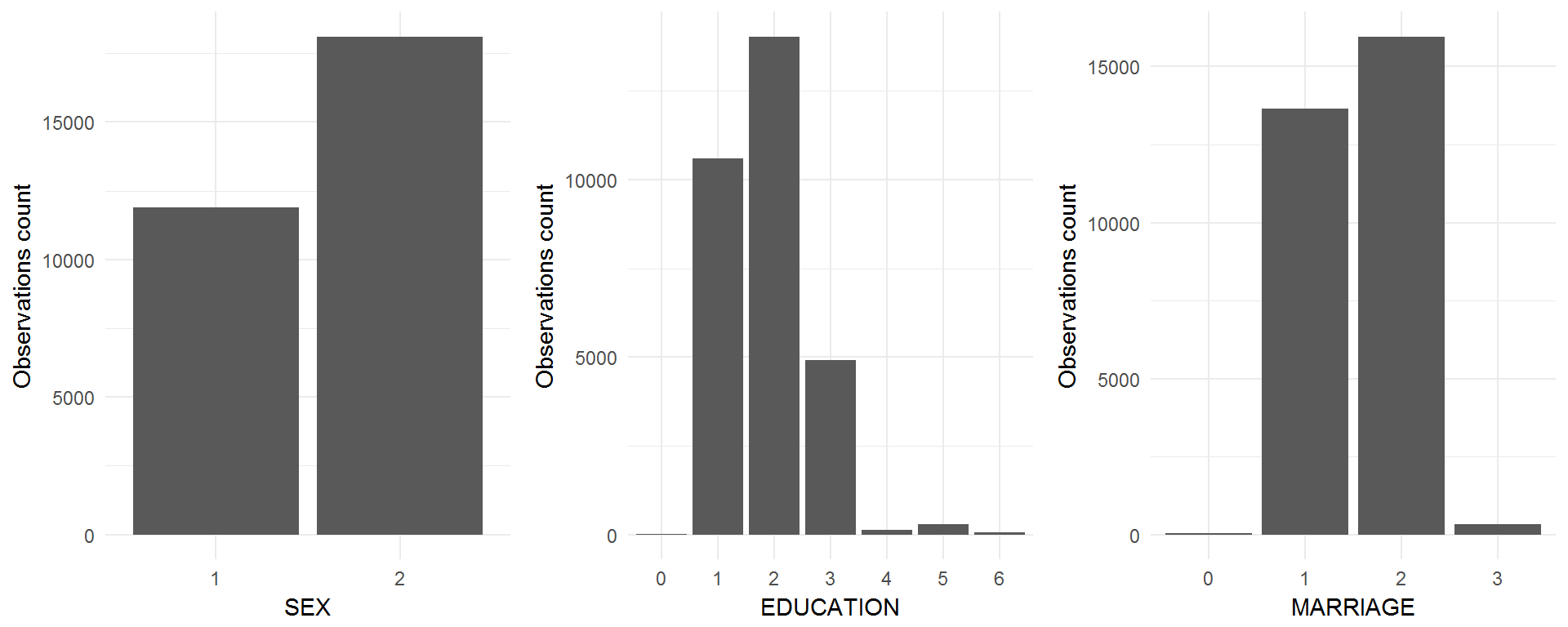# Introduction

This report contains two parts devoted to Exploratory Analysis and Prediction Models. In the chapters, I tried to touch on the most popular methods and algorithms in order to find the best model which will help predict default and to answer the questions:

1. How does the probability of default payment vary by categories of different demographic variables?
2. Which variables are the strongest predictors of default payment?

I hope you will enjoy this report.

In this research, I use Google’s R Style Guide

# Data

## Data Overview

This dataset contains information on default payments, demographic factors, credit data, history of payment, and bill statements of credit card clients in Taiwan from April 2005 to September 2005. The data set comes from the UCI Machine Learning Repository Irvine, CA: University of California, School of Information and Computer Science.

Number of Instances: 30000

## Data Dictionary

There are 25 variables:

• ID: ID of each client
• LIMIT_BAL: Amount of given credit in NT dollars (includes individual and family/supplementary credit)
• SEX: Gender (1=male, 2=female)
• EDUCATION: (1=graduate school, 2=university, 3=high school, 4=others)
• MARRIAGE: Marital status (1=married, 2=single, 3=others)
• AGE: Age in years
• PAY_0: Repayment status in September, 2005 (-1=pay duly, 1=payment delay for one month, 2=payment delay for two months, … 8=payment delay for eight months, 9=payment delay for nine months and above)
• PAY_2: Repayment status in August, 2005 (scale same as above)
• PAY_3: Repayment status in July, 2005 (scale same as above)
• PAY_4: Repayment status in June, 2005 (scale same as above)
• PAY_5: Repayment status in May, 2005 (scale same as above)
• PAY_6: Repayment status in April, 2005 (scale same as above)
• BILL_AMT1: Amount of bill statement in September, 2005 (NT dollar)
• BILL_AMT2: Amount of bill statement in August, 2005 (NT dollar)
• BILL_AMT3: Amount of bill statement in July, 2005 (NT dollar)
• BILL_AMT4: Amount of bill statement in June, 2005 (NT dollar)
• BILL_AMT5: Amount of bill statement in May, 2005 (NT dollar)
• BILL_AMT6: Amount of bill statement in April, 2005 (NT dollar)
• PAY_AMT1: Amount of previous payment in September, 2005 (NT dollar)
• PAY_AMT2: Amount of previous payment in August, 2005 (NT dollar)
• PAY_AMT3: Amount of previous payment in July, 2005 (NT dollar)
• PAY_AMT4: Amount of previous payment in June, 2005 (NT dollar)
• PAY_AMT5: Amount of previous payment in May, 2005 (NT dollar)
• PAY_AMT6: Amount of previous payment in April, 2005 (NT dollar)
• default.payment.next.month: Default payment in June, 2005 (1=yes, 0=no)

## Importing Data

Let’s load the data, remove some extra data and make basic transformations:

``````library(XLConnect)
data.file.path <-
file.path(getwd(), "Data", "default of credit card clients.xls")

raw.data <-
startCol = 1,
startRow = 2,  # first row is synthetic names such as X1
autofitCol = TRUE)

# Converting columns to factors according to the data description
pay.cols.names  <- paste0("PAY_", c(0, 2:6))  # "PAY_0" "PAY_2" "PAY_3"...
cols.to.factors <- c("SEX", "EDUCATION", "MARRIAGE",
pay.cols.names, "default.payment.next.month")
raw.data[cols.to.factors] <- lapply(raw.data[cols.to.factors], factor)``````

# Exploratory Data Analysis

## Exploration of Predictors

#### Categorical

##### Repayment Status (PAY_X)

Let’s compare the documentation provided for the data and a real situation. According to the description, this PAY_X is a set of categorical variables with the levels: -1=pay duly, 1=payment delay for one month, 2=payment delay for two months, … 8=payment delay for eight months, 9=payment delay for nine months and above. Let’s look at real PAY_X columns:

``````library(ggplot2)
# Generating bar plots for PAY_0..6 variables
pay.histograms <-
lapply(pay.cols.names,
function (pay.col.name){
ggplot(data = raw.data[, pay.cols.names],
aes(x = raw.data[, pay.col.name])) +
geom_bar(stat = "count") +
theme_minimal() +
xlab(paste0("Repayment status ", pay.col.name)) +
ylab("Observations count")
})
MultiPlot(plotlist = pay.histograms, cols = 3)``````We observe undocumented values for repayment status variables: `-2` and `0`. Moreover, fraction of it is `86.5%.` Strictly speaking, it is “NAs”. Due to this, there are several standard strategies to deal with:

• Remove observations with NAs. After that we will have 4061 from 30000 observations. Obviously, the loss is too great.
• Delete variables. I would suggest exploring the prediction power of this before removing these variables.
• Replace NAs by major class. But our NA is a major class already. Additionally, it does not look like NAs are random. So, this is a wrong approach in our case.
• Keep this data as an “NA classes”. I think this is the best decision at this stage of the research and we should keep this data “as is”.
##### Social Status Predictors (SEX, EDUCATION, MARRIAGE)
``````# Getting social predictors only
dem.raw.data <- raw.data %>% select(SEX:MARRIAGE)

# Generating bar plots for PAY_0..6 variables
dem.histograms <-
lapply(colnames(dem.raw.data),
function (pay.col.name){
ggplot(data = raw.data[, pay.cols.names],
aes(x = raw.data[, pay.col.name])) +
geom_bar(stat = "count") +
theme_minimal() +
xlab(pay.col.name) +
ylab("Observations count")
})
MultiPlot(plotlist = dem.histograms, cols = 3)``````We have some undocumented value `0` for `EDUCATION` and `MARRIGE`. I suggest this as `NA`. Also, let’s rename levels SEX to `Male` and `Female`

``````library(plyr)
# Renaming Male/Female levels for SEX variable
levels(raw.data\$SEX)[levels(raw.data\$SEX) == "1"]             <- "Male"
levels(raw.data\$SEX)[levels(raw.data\$SEX) == "2"]             <- "Female"
str(raw.data\$SEX)``````
``##  Factor w/ 2 levels "Male","Female": 2 2 2 2 1 1 1 2 2 1 ...``

#### Numerical

##### Age (AGE)
``````# Getting ID and AGE columns only
age.raw.data <- raw.data[c("ID", "AGE")]

# Calculate a cutoff for the "rule of thumb"
age.thumb.cutoffs <-
apply(age.raw.data, 2,
function (t){
Q3 = quantile(t, .75) + 1.5 * IQR(t)
}
) %>% t()``````
``````# Generating a histogram for the AGE variable with a vertical cutoff line
age.hist.plot <-
ggplot(data = age.raw.data,
aes(x = age.raw.data\$AGE)) +
geom_histogram(binwidth = 1,
colour = "black", fill = "white") +
geom_vline(aes(xintercept = age.thumb.cutoffs[1, "AGE"]),
colour = "#BB0000", linetype = "dashed") +
theme_minimal() +
xlab("Age")

# Generating a scatterplot for the AGE variable with a horizontal cutoff line
age.scatter.plot <-
ggplot(data = age.raw.data,
aes(x = 1:length(age.raw.data\$AGE),
y = age.raw.data\$AGE)) +
geom_point(shape = 1,
position = position_jitter(height = .5)) +
geom_hline(aes(yintercept = age.thumb.cutoffs[1, "AGE"]),
colour = "#BB0000", linetype = "dashed") +
theme_minimal() +
xlab("Index") + ylab("Age")

# Printing both plots near each other
MultiPlot(age.hist.plot, age.scatter.plot)``````